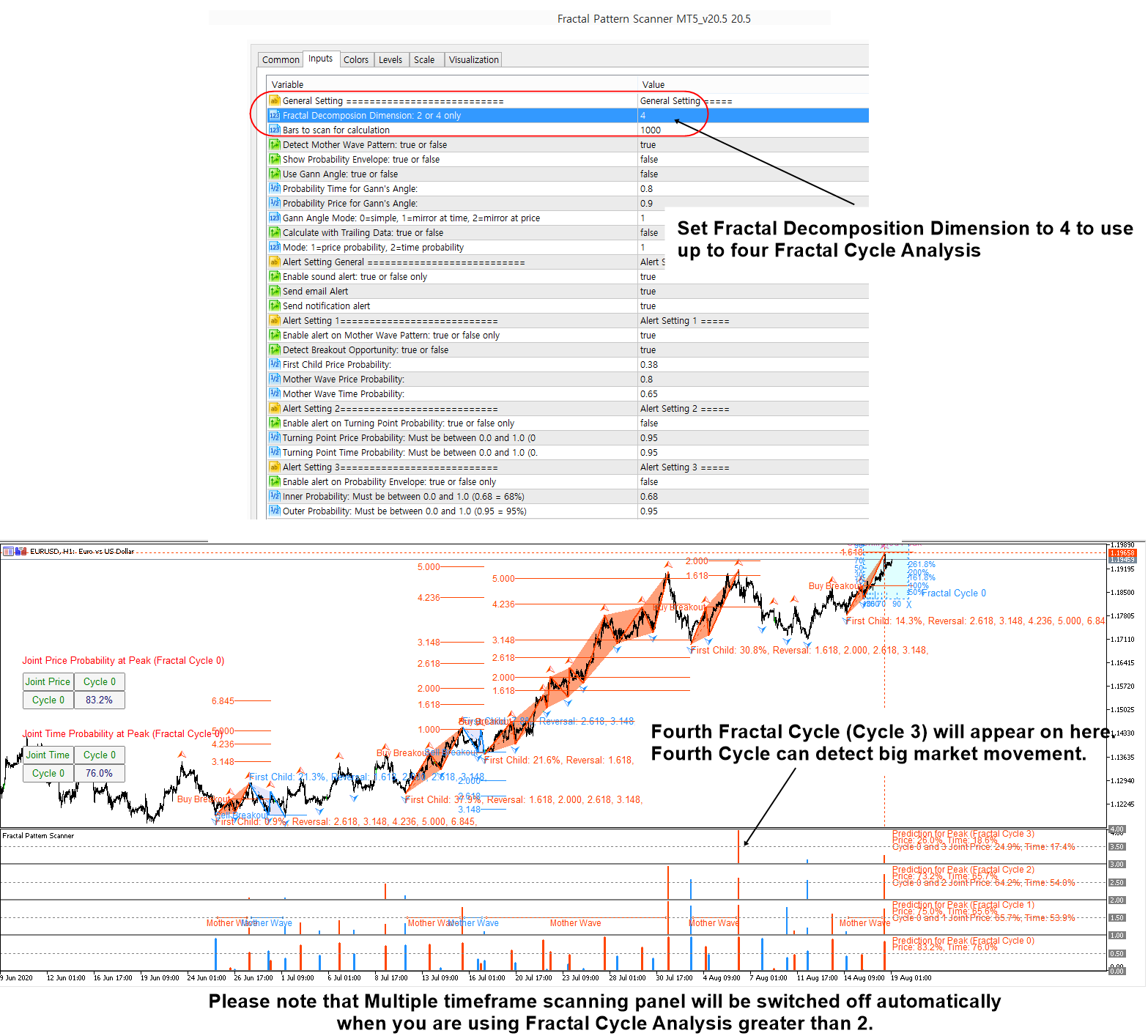# Fractal Cycle with Fractal Pattern Scanner

In terms of the Financial trading point of view, the Forex and Stock market are recognized to have stochastic cycle rather than deterministic cycle. Stochastic cycle in Forex and Stock market means that we, including financial traders and average people, feel like that market is repeating but not with the exact time interval. For example, many scientist will say that the US business cycle will repeat in every 5 to 10 years but they will never say that the US business cycle will repeat in every 5 years definitely. That is exactly the meaning of the stochastic cycle. Stochastic cycle means we can not fix the time interval for the market. From the Financial trading point of view, the accurate prediction of the market direction really depends on how much you understand the stochastic cycle existing in the Forex and Stock market.

In order to model stochastic cycle, we need to map the price series into geometric space. This can be done using Peak Trough transform. In theory, we could perform cycle analysis on several different ways. For example, we can model the largest fractal wave first. Then we can break down the largest cycle to smaller cycle. We can continue this process until we have reasonably smaller cycle. This is the top down approach. On the other hands, we can start to model extremely smaller cycle first. Then, we can combine several small cycles to form the bigger cycle. We can continue until we have the largest fractal cycle. This is the bottom up approach. This sort of Fractal Decomposition analysis is considered as Fractal Cycle Analysis (FCA). Fractal Cycle Analysis is the stepwise refinement of the Fractal Decomposition analysis for the purpose of your application. In the application of the financial trading, the stepwise refinement can be done to catch both micro and macro movement of the market.

To aid your understanding, we will bring the counterpart example in the case of deterministic cycles because it is easier to visualize the concept this way. For example, in many ways, the Fractal Cycle Analysis (FCA) resembles the Singular Spectrum Analysis (SSA), which models deterministic cycles in time series. For example, both FCA and SSA are designed to model the cycles in multiple scales. FCA model stochastic cycles whereas SSA model deterministic cycles in the data.

Fractal Pattern Scanner provides up to four Fractal Cycle analysis using Fractal Decomposition technique. In default setting, Fractal Pattern Scanner is set to do two Fractal Cycle analysis. The default setting will provide the Fractal Cycle analysis for Cycle 0 and Cycle 1.

When you set Fractal Decomposition Dimension = 4, you can access four cycles including Cycle 0, Cycle 1, Cycle 2 and Cycle 3.

Cycle 0 is smallest cycle and Cycle 3 is the biggest cycle. Cycle 0 and Cycle 1 are useful for day trading. Cycle 2 and Cycle 3 can be used to analyze any big movement in the financial market.

For your information, when you set Fractal Decomposition Dimension = 4, multiple timeframe pattern detection panel is not available because Mutltiple timeframe pattern detection panel is only available with two Fractal Cycle analysis (Fractal Decomposition Dimension = 2).

Occasionally, you will get only three Fractal Cycles in the output. It is because the algorithm will get rid of any insignificant cycle from the analysis. Do not get surprise even if you get the three cycles only even if you have set Fractal Decomposition Dimension = 4.

Here are the landing page to Fractal Pattern Scanner for MetaTrader 4 and MetaTrader 5 platform.

https://www.mql5.com/en/market/product/49170

https://www.mql5.com/en/market/product/49169